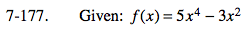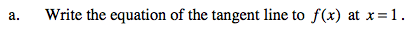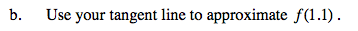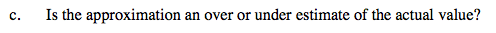### Home > CALC > Chapter 7 > Lesson 7.4.1 > Problem7-177

7-177.Use point-slope form of a line. f(1) will give you the point, and f '(1) will give you the slope.Since a linear equation is easier to evaluate than a 4th-degree polynomial, use the tangent line to approximate f(1.1).Is the graph of y = f(x) concave up or down x = 1?